# 630 Factor Trees and Level 2

### Today’s Puzzle: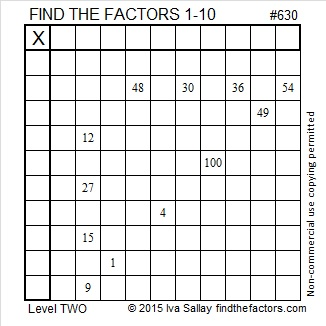Print the puzzles or type the solution on this excel file: 10 Factors 2015-09-28

### Factors for Today’s Puzzle: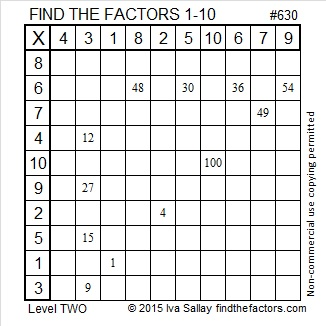### Factor Trees for 630:

630 is the 7th number with exactly 24 factors. So far, the seven numbers counting numbers with 24 factors are 360, 420, 480, 504, 540, 600, and 630. No counting number less than 630 has more than 24 factors.

Two of those seven numbers make up the Pythagorean triple 378-504-630. Which factor of 630 is the greatest common factor of those three numbers in the triple?

Here are a few of the MANY possible factor trees for 630.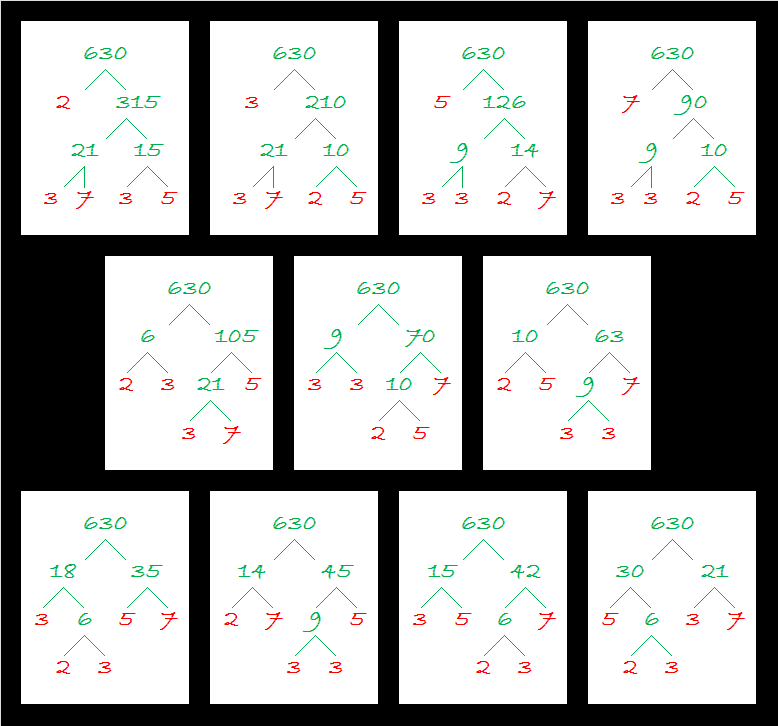### Factors of 630:

• 630 is a composite number.
• Prime factorization: 630 = 2 x 3 x 3 x 5 x 7, which can be written 630 = 2 x (3^2) x 5 x 7
• The exponents in the prime factorization are 1, 2, 1, and 1. Adding one to each and multiplying we get (1 + 1)(2 + 1)(1 + 1)(1 + 1) = 2 x 3 x 2 x 2 = 24. Therefore 630 has exactly 24 factors.
• Factors of 630: 1, 2, 3, 5, 6, 7, 9, 10, 14, 15, 18, 21, 30, 35, 42, 45, 63, 70, 90, 105, 126, 210, 315, 630
• Factor pairs: 630 = 1 x 630, 2 x 315, 3 x 210, 5 x 126, 6 x 105, 7 x 90, 9 x 70, 10 x 63, 14 x 45, 15 x 42, 18 x 35, or 21 x 30
• Taking the factor pair with the largest square number factor, we get √630 = (√9)(√70) = 3√70 ≈ 25.09980.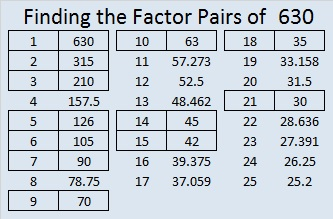### Sum-Difference Puzzle:

630 has twelve factor pairs. One of the factor pairs adds up to 53, and a different one subtracts to 53. If you can identify those factor pairs, then you can solve this puzzle!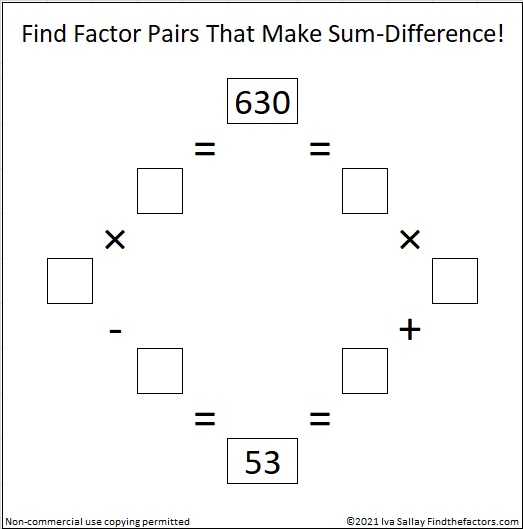### More about the Number 630:

630 is the sum of the six prime numbers from 97 to 113.

630 is the 35th triangular number because (35 x 36)/2 = 630. It is also the 18th hexagonal number because 18(2 x 18 – 1) = 630.

630 is a triangular number that is a multiple of other triangular numbers in more ways than you probably want to know:

• 630 is three times the 20th triangular number, 210, because 3(20 x 21)/2 = 630.
• 630 is 6 times the 14th triangular number, 105, because 6(14 x 15)/2 = 630.
• 630 is 14 times the 9th triangular number, 45, because 14(9 x 10)/2 = 630.
• 630 is 30 times the 6th triangular number, 21, because 30(6 x 7)/2 = 630.
• 630 is 42 times the 5th triangular number, 15, because 42(5 x 6)/2 = 630.
• 630 is 63 times the 4th triangular number, 10, because 63(4 x 5)/2 = 630.
• 630 is 105 times the 3rd triangular number, 6, because 105(3 x 4)/2 = 630.
• 630 is 210 times the 2nd triangular number, 3, because 210(2 x 3)/2 = 630.
• and finally, 630 is 630 times the 1st triangular number, 1, because 630(1 x 2)/2 = 630.